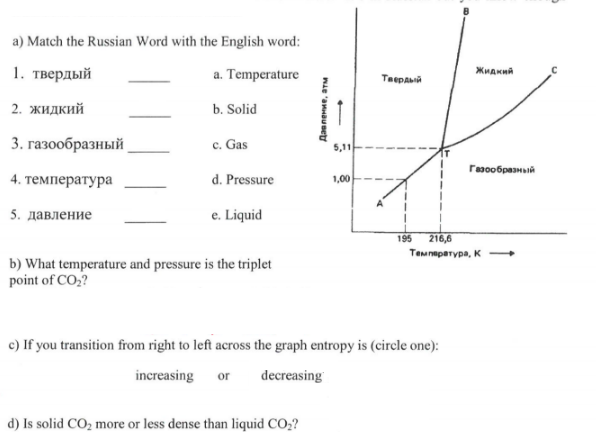# Problem: The phase diagram of CO2 is shown here. It's in Russian but you know enough science to be able to translate the words.

###### FREE Expert Solution
91% (339 ratings)
###### Problem Details

The phase diagram of CO2 is shown here. It's in Russian but you know enough science to be able to translate the words.What scientific concept do you need to know in order to solve this problem?

Our tutors have indicated that to solve this problem you will need to apply the Phase Diagram concept. You can view video lessons to learn Phase Diagram. Or if you need more Phase Diagram practice, you can also practice Phase Diagram practice problems.

What is the difficulty of this problem?

Our tutors rated the difficulty ofThe phase diagram of CO2 is shown here. It's in Russian but ...as medium difficulty.

How long does this problem take to solve?

Our expert Chemistry tutor, Dasha took 4 minutes and 42 seconds to solve this problem. You can follow their steps in the video explanation above.

What professor is this problem relevant for?

Based on our data, we think this problem is relevant for Professor Ratliff's class at USF.